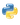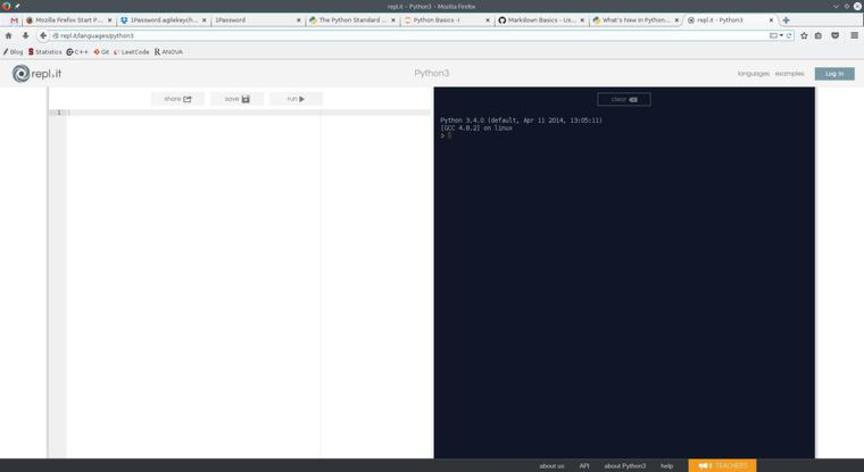# Python Tutorial - Week 1

Python is a widely used general-purpose, high-level programming language. Due to its focus on readability, wide-spread popularity and existence of a plethora of libraries (also called modules), it is one of the most preferred programming languages for scientists and engineers.

## Python for Non-Programmers

In this series of python tutorials, I will provide a set of lectures on various basic topics of python. The prime target audience for this series are scientist in non-programming fields like microbiology, genetics, psychology etc. who have some to none programming experience.

Here is a brief list of topics I will cover per week. I will also post exercises at the end of each session, along with the expected outputs. You should plan to complete these exercises within 5-6 days, before the new tutorial is posted. You will be judging your exercises on your own. The goal should be to match your program's output to the expected output.

• Week 1 : Working with Python on Windows, Concept of Variables & Math Operations, Displaying Outputs
• Week 2 : User Inputs, Modules, Comments and Basics of Strings
• Week 3 : More on Strings, Lists and Other Containers
• Week 4 : Looping/iterating, if/else Conditions
• Week 5 : Advanced String Operations
• Week 6 : Regular Expressions and Strings
• Week 7 : Reading and Writing Files
• Week 8 : Functions and Writing Scripts
• Week 9 : Interacting with Operating System
• Week 10 : Handling and Plotting Data in Python
• Week 11 : Basic Statistics in Python using Pandas
• Week 12 : Introduction to BioPython

### Week 1. Introduction to Python

To start working with any programming language, first thing you need is a working installation of that language. Today, we will go through installation of python on Windows machines.

To keep things simple, We will be running our simple programs in google chrome browser, without any need of an installation.

For later exercises, from Week 7 onwards, I would highly recommend getting access to a Linux/Mac machine. However, I will also provide doing the same things on Windows machines and Google Chrome as well.

In this week's session, I will be assuming you will be using online python in google chrome.

To follow these tutorials, please run any code and observe output that you see in code blocks.

#### Installing Python

Go to the following website to get access to python.

You should get a window like this:You can work with this system in two ways: A. Write your code interactively (one command at a time) on the dark screen on the left. Pressing ENTER will show you the output of that particular command.

Lets' try our first program:

In :
print("My Name is so-and-so")

My Name is so-and-so


The above program will simply output whatever was put under "quotes". We will learn more about the print() method (what is a method/function in python?) towards the end of this session.

### Variables¶

A variable is a symbol or name that stands for a value. For example, in the expression x = 23

x is a name/symbol whose value is numerical and equal to 23. if we write something like this: y = x+12-5, then y is a new variable whose value should be equal to 23+12-5.

Let's try these in a program

In :
x=23
print("x = ",x)

x =  23

In :
y=x+12-5
print("y =",y)

y = 30

In :
print(23+12-5)

30


We ran 3 commands. First we created a variable 'x' with a value of 23. We verified it value by using a print() method! Second, we created another variable called 'y' whose values is equal to mathematical operation of 'x+12-5'. If you remember basic algebra, this is exactly that. Finally, we confirmed that value of 'y' is exactly equal to 23+12-5.

Hopefully, you got a feel of variables. Variables are not limited to just numbers though. We can also store text. For example:

In :
name = "Sadanand Singh"
print("My Name is:", name)

My Name is: Sadanand Singh


Here, we saved my name Sadanand Singh in a variable called "name".

Concept of variable is very fundamental to any programming language. You can think of them as tokens that store some value.

Lets consider this example to understand variable in more detail.

You are doing your taxes, all of your calculations depend on your net income. If you do all your calculation in terms of actual value of net income, every time you write the number, your chances of making a mistake increases. Furthermore, suppose, you want to update the income due to some mistake in the beginning, now you have update every place where you used your income.

Lets consider a second case where you declare a variable called "income", and store income = 30000. Now, any time you do any calculation with income, you will be using the variable "income". In this framework, because you have to type your income just once, chances of making mistakes are least and changing it needs just one change!

### Math Operations

Now that we know how to declare and use variables, we will look at first what all we can do with numbers in python. Using simple math operations are extremely easy in Python. Here are all the basic math operations that one can do in Python.

SyntaxMathOperation Name
a+b$a + b$Addition
a-b$a - b$Subtraction
a*b$a \\times b$Multiplication
a/b$a \\div b$Division
a**b$a^b$Power/Exponent
abs(a)$\\lvert a \\rvert$Absolute Value
-a$-1 \\times a$Negation
a//bquotient of $a \\div b$Quotient
a%bRemainder of $a \\div b$Remainder

Here are some example operation. Please repeat these and observe the use of parenthesis in using the BODMAS principle.

In :
3 + 5

Out:
8
In :
2 * 3 + 3.34 + 4 - 45.67

Out:
-32.33
In :
12.7 - 10 * 23.5 / 0.5

Out:
-457.3
In :
12 - (11 + 34) / 2

Out:
-10.5
In :
a,b=5,6


Above is a special case of assigning multiple variables together. In the above example we stored a=5 and b=6. Lets confirm these:

In :
print("a = ",a)
print("b = ",b)

a =  5
b =  6

In :
c = a**b
print(" a raised to the power b is:",c)

 a raised to the power b is: 15625

In :
b = -b

In :
print("b = ",b)

b =  -6


Can you guess what we did here? First we use negation operation to get "-b" i.e. -6. Then, we we redefined b to be equal to -6! Let consider the following example: a = a-b-3. What do you expect the value to be? Now lets check if you are correct:

In :
a = a-b-3
print("new value of a is: ",a)

new value of a is:  8


What do you expect if we do b = -b again?

In :
b = -b
print("New Value of b is:",b)

New Value of b is: 6

In :
a//b

Out:
1
In :
a%b

Out:
2
In :
b**-2

Out:
0.027777777777777776

You can perform many other advanced math operations using the "math module". To use them, first you will need to import the math module in your code like this:

In :
import math


Then, you use operations like the following:

In :
c = math.sqrt(25)
print(c)
c = math.log(10)
print(c)
c = math.log10(10)
print(c)
c = math.cos(math.pi)
print(c)

5.0
2.302585092994046
1.0
-1.0


You can all other available mathematical operation in this module on the python website.

Primary way to see and print information on your screen in python is using a method/function called print(). We will learn more about functions in python later. For today, you can think of functions as a program that "does something". For example, the function print(), does the job of printing things on screen.

Notice the use of () in functions. () separates a function from a variable. For example, "foo" is a variable, whereas foo() is a function, sometimes also called as method.

Functions also have a concept of arguments. Arguments can be thought as inputs to functions. For example, we have function that adds 2 numbers, then this function will need 2 arguments, the two numbers that we want to add. We can denote this function as, addition(a,b).

Similarly, functions also have a concept of return values. Return value can be thought as the output of that function. For example, in the above example of addition(a,b) function, sum of two numbers will be the "return value" of the function. We can write this as, c = addition(a,b). Here, a and b are arguments to function addition() and c is the return value of this function.

A function can have any number of arguments, zero to any number; where it can have either zero or 1 return values.

Now, coming back to the print() method, that we have been using throughout this tutorial.

print() method can take any number of arguments separated by commas. All it does is to "print" those on your screen. Lets look at some examples:

In :
print(3,4)
print("My Name is Sadanand Singh")
print("My Name is ","Sadanand Singh","and My age is: ", 29)

3 4
My Name is Sadanand Singh
My Name is  Sadanand Singh and My age is:  29


Now, lets try something fun.

In :
print("My Name is","Sadanand", sep="***")

My Name is***Sadanand

In :
print("My Name is","Sadanand","Singh","My Age is","12","",sep="***")

My Name is***Sadanand***Singh***My Age is***12***


Can you explain what is happening here!

## Excercise

We will all things we have learned today using the exercise below.

We will follow the tax preparation example:

1. Create a variable to store "income"
2. Create another variable called "taxRate" which is equal to 1/100000th of "income".
3. Net federal tax will be equal to 1.5 times income times taxRate
4. Net state tax will be equal to square root on federal tax
5. Net tax will be federal tax + state tax
6. Total final tax will be Net tax + log to the base of 2 of Net Tax
7. Print following values clearly using print(): income, taxRate, Federal Tax, State Tax, Net Tax and Final Tax.
8. First run with an income of 60000
9. Repeat with an income of 134675

Your output should look like the following in two cases.

Case 1: income = 60000

1. Total Income is: 60000
2. Tax Rate is: 0.059999999999999998
3. Total Federal Tax is: 1800.0
4. Total State Tax is: 42.426406871192853
5. Net Tax is: 1842.4264068711927
6. Total Tax is: 1853.2737981499038

Case 2: income = 134675

1. Total Income is: 134675
2. Tax Rate is: 0.13467499999999999
3. Total Federal Tax is: 9068.6778125000001
4. Total State Tax is: 95.229605756298284
5. Net Tax is: 9163.9074182562981
6. Total Tax is: 9177.0691654243201

Great! Next week we dive into Python further.

Share This:

## Comments

### Stay in touch

Like the posts you see here? Sign up to get notified about new ones.

# Get The Best Of Posts Delivered To Your Inbox

Subscribe to my newsletter and stay updated.